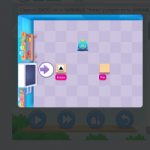Smartick is a fun way to learn math!Jun24

## What Are Boolean Operators and Their TypesRobby’s laboratory is an activity within Smartick Coding that is used to teach concepts about the use of variables, input, output, and different types of data in programming. In the laboratory, we can find data that are numbers, shapes, or letters, but we can also find new data there, such as Boolean operators. What are […]

May05

## Half, Third, Fourth, Fifth in Math: Definition & CalculationIn this post, we are going to learn how to calculate a half, third, fourth, and fifth. These expressions are not just used for math problems, but also in daily life! Do you know what they are? Do you know how to calculate them? You are going to see how easy it is to calculate […]

Apr21

## Subtraction With and Without CarryingToday we are going to learn how to solve subtraction problems both with and without carrying. We will begin with a review of what subtraction is and its elements, and then we will see an example of each type of subtraction with a video. What is Subtraction? Subtraction is a mathematical operation that consists of […]

Apr13

## What is Multiplication: Steps to Learn and Understand Multiplication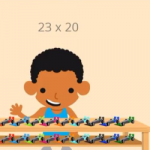What is multiplication? We will explain it in this post! Then we will show some examples using the steps that you should follow to complete multiplication problems, along with a video. What is Multiplication? Multiplication is a mathematical operation that is performed to calculate the result by adding the number as many times as indicated […]

Mar25

## Examples of 3rd Grade Math Word Problems with SolutionsIn this post, we are going to look at a selection of 3rd grade math word problems in Smartick. We’ll begin by explaining what a word problem is. What is a Word Problem? A word problem is a question or approach, that cannot be answered using one specific operation, but rather a combination of previous […]

Mar11

## Consecutive Interior Angles: What They Are and How to Find Them With Examples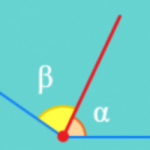In this post, we are going to discover what consecutive interior angles are and how to differentiate them from other types of angles. You will see some examples and even a little quiz. But before we start, let’s review what an angle is. An angle is the portion of a plane between two rays that […]

Feb04

## Examples of Second Grade Word Problems with Solutions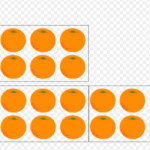In today’s post, we are going to see a selection of Second Grade word problems that usually appear at the end of a Smartick session for children with a second-grade level. You will also get a chance to see the solution for each problem. Ready? Let’s go! How To Correctly Solve Any Problem Read the word […]

Jan15

## What is an Obtuse Angle?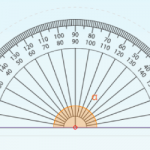In today’s post, we are going to learn about what an obtuse angle is with different examples taken from everyday life and some Smartick exercises. What is an Obtuse Angle? An angle is a space shared by two rays that are joined at the same vertex. We can find different types of angles according to […]

Dec28

## Complementary Angles: What Are They?In today’s post, we are going to learn about what complementary angles are and their properties. After, we will look at some examples and complete a few exercises together. Before we begin, we would recommend reviewing the types of angles that exist. What Are Complementary Angles? Two angles are complementary if the sum of the […]

Nov26

## Perimeter: What Is It and How to Find It for Any Polygon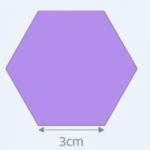Today we are going to learn to calculate perimeters of geometric figures. But first, let’s start with the definition. What is a Perimeter? A perimeter is what we call the length of the outline of a geometric figure. Therefore, the perimeter is a measurement of length, so it will come in centimeters, inches, and feet, […]

Oct30

## What is a Straight Angle and ExamplesIn today’s post, we will learn what a straight angle is, how many degrees it has, and its properties. We will also practice with some examples. What is a Straight Angle? An angle is a portion of the plane between two rays that are joined by a vertex. A straight angle is the shared space […]